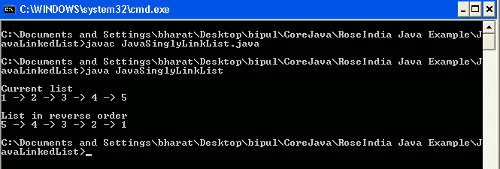Tutorials

In this section we will discuss about the own implementation of Linked List in Java.

In this section we will discuss about the own implementation of Linked List in Java.

In this section we will discuss about the own implementation of Linked List in Java.

Linked List is a part of Data Structure. In Linked List nodes are connected by a link this link is referred to as one node contains the reference of next node. In this tutorial we will learn this by a simple example. In this example we will display an original list and then display its reverse order.

Example

Here I am going to give a simple example which demonstrate how nodes are linked to each other and how can we displayed in their order as well as in its reverse order. In this example I have created Java classes. The Java class Link instantiates a reference variable next which will be used for linking the next value in the list. Then created a class named LinkList where written methods for printing the list value in its order and its reverse order. Then created a main class where created the list values and called methods for displaying list values.

Source Code

```class Link
{
String num;
{
this.num = num;
}
public String toString()
{
return num;
}
{
{
System.out.print (node);
if (node.next!=null)
{
System.out.print (" -> ");
print(node.next);
}
}
public void reverseList()
{
while (node3!= null)
{
node1 = node2;
node2 = node3;
node3 = node2.next;
node2.next = node1;
}
first = node2;
}
{
public static void main (String args[])
{
l1.next = l2;
l2.next = l3;
l3.next = l4;
l4.next = l5;
LL.first = l1;
System.out.println ("\nCurrent list");
LL.print(LL.first);
LL.reverseList();
System.out.println();
System.out.println ("\nList in reverse order");
LL.print(LL.first);
System.out.println();

}
}

```

Output

When you will execute the JavaSinglyLinkList.java then the output will be as follows :